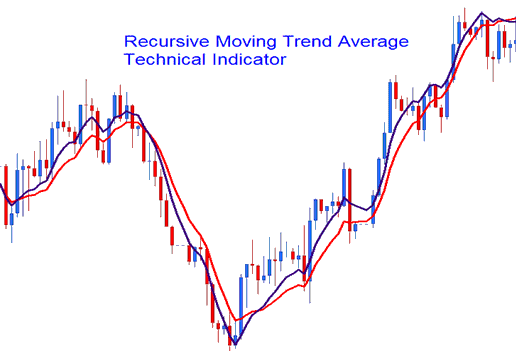# Recursive Moving Indices Trend Average Indices Technical Analysis and MT5 Stock Indexes Indicator

This MetaTrader 5 Indices Indicator is calculated using a mathematical polynomial fit, the formula is referred as the Recursive Moving Polynomial Fit.

This formula used to calculate this MetaTrader 5 technical indicator only requires a small set of previous data to calculate and predict the next direction of stock indexes price movement. The example explained and illustrated below shows two Recursive Averages combined to form a crossover stock indexes trading system method.## Indices Technical Analysis and Generating Indices Trading Signals

The best technical analysis method is the crossover method where you can combine two recursive averages, such as the 14 and 21. When the two crossovers each other upwards then that is a bullish signal while a downward crossover is a bearish signal.The Recursive Average looks similar to the traditional moving average, the only difference is that is much smoother due to the method of calculation that it uses and much less prone to whipsaws.